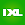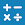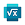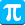# Maple Calculator: Math Solver

by Maplesoft
1,000,000+ installs

Android application Maple Calculator: Math Solver developed by is listed under category Education. According to Google Play Maple Calculator: Math Solver achieved more than 1,000,000 installs. Maple Calculator: Math Solver currently has 5,606 ratings with average rating value of 4.28. The current percentage of ratings achieved in last 30 days is 7.82%, percentage of ratings achieved in last 60 days is 20.03%. Maple Calculator: Math Solver has the current market position #55656 by number of ratings. A sample of the market history data for Maple Calculator: Math Solver can be found below. Last update on 2021-12-05.

Title: Maple Calculator: Math Solver Maplesoft Education Free Android
Total ratings: 5606 7.82% 20.03% 4.28
Installs (achieved): 1,000,000+ 2,557,481
5 star ratings: 3,933 605 437 0 605

2021-04-17: Android application Maple Calculator: Math Solver achieved 1,000,000 installs. Android application Maple Calculator: Math Solver achieved 500,000 installs. Android application Maple Calculator: Math Solver achieved 100,000 installs. Android application Maple Calculator: Math Solver achieved 50,000 installs. Android application Maple Calculator: Math Solver achieved 10,000 installs.

Total number of ratings
Total number of active users rated for Maple Calculator: Math Solver.

Total number of installs (*estimated)
Estimation of total number of installs on Google Play. Approximated from number of ratings and install bounds achieved on Google Play.

Average rating
Average rating value on Google Play. Given by active users of the application.